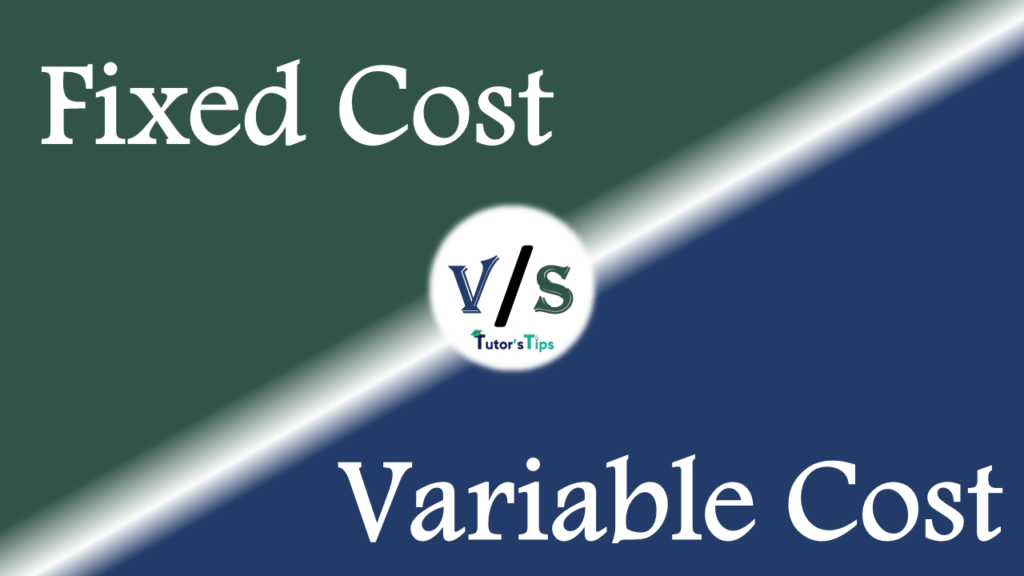# Difference between Fixed Cost and Variable CostThe basic difference between Fixed Cost and Variable Cost is that Fixed cost refers to the expenditure incurred on fixed factors of production and Variable cost refers to the cost incurred on variable factors of production.

To know the difference between these two, we must clear the meaning of these terms:

## Meaning of Fixed Cost:-

It represents the expenditure incurred on the use of fixed factors of production. Total Fixed cost can be calculated as:

TFC = Units of fixed factors used × Price of Factor

These are the costs which do not change with the change in the output. In the short term, these costs do not vary with the level of output. Therefore,  It remains the same whether the output is zero or maximum. These are also known as supplementary costs, overhead costs, historical costs, Unavoidable costs or indirect costs.

## Meaning of Variable Cost:-

It refers to the cost which is incurred on variable inputs of production. These are the costs which change with the change in the output. Thus, It means when the output increases, the variable costs increase and it decreases with the fall in output. Also, this cost falls to zero when there is no output. These costs are also known as Prime costs or Direct costs or Avoidable Costs.

Some of the examples of Variable Costs are:

1. Expenditure on purchase of raw material
2. Wages to labour
3. Expenditure on electricity
4. Wear and tear Expenses

## Chart of Difference between Fixed Cost and Variable Cost :

 Basis of Difference Fixed Cost Variable Cost Meaning It refers to the expenditure incurred by the producer on fixed factors of production. It refers to the cost incurred on variable factors of production. Nature Fixed Costs are time-based costs as these are fixed in short-run and can be variable in long run. Variable Costs are volume of production based as these are dependent on the units produced. Behaviour These costs are fixed and don’t change with increase or decrease in the output. These costs are variable. Thus, keep on changing with the change in output. Incurred These costs are incurred even before the start of actual output. These costs are incurred only when there is the actual output produced. Unit Cost The per-unit fixed cost decreases with an increase in production and vice versa. Thus, it changes when comes to per-unit cost. The per-unit variable cost is always fixed whatever be the production. Control These costs cannot be controlled and have to be paid. These costs can be controlled by controlling the volume of production. Also known as These costs are also known as supplementary costs, overhead costs or indirect costs. These costs are also known as Prime cost or direct cost. At zero production level These costs have to be incurred even when the output is zero. These costs are zero when the production is zero. Examples Expenditure on Machinery and Plant, Licence fee, Expenditure on Land and Building etc. Expenditure on purchase of raw material, Wages to labour, Expenditure on electricity, Wear and tear Expenses etc.

## Conclusion:

Thus, these both costs stand for every business enterprise. The thing which makes these both distinct is the consistency and stability of costs with a period of time and the production level. Fixed costs are fixed for a specific period of time whereas the variable costs vary with the production.